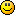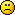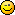# Game of 24

Jump to Last Post 1-16 of 16 discussions (41 posts)
1. Welcome Aboard!

Here are the rules to the game:

1. You are given 4 numbers, e.g. 1234 and must use each number, only once, to make 24
2. You may use addition, subtraction, multiplication & division as many times as you want
3. Once you've solved the problem from the post above, make your own by writing your 4 digits (you can only use numbers from & including 0-9

Note: There may be more than one solution to a problem. Feel free to post as many as you can think of!

Example: Use 1234 to make 24.
(4+2) x (3+1) = 24!

-----------------------------------------------------------------

First problem below:

Make 24 using the numbers "6371"

1.0
Ben Blackwellposted 8 years agoin reply to this

Sounds interesting.
6 * 3 + 7 - 1 = 24

1. nice Ben! Now post a problem of your own2.0
Ben Blackwellposted 8 years ago

New problem: 9402

3. Tough one Ben! What's your solution? Is square root acceptable in this situation?

Can I do √9 x (4 x 2) + 0 = 24?

1. You cannot use square roots, sorry, however I'm not sure how you would do without it. Well done nonetheless for solving this one Brycekiguchi!

Ben, do you have a solution that doesn't involve using square roots?

4. If there are no objections, here's the next one: 9876

1.0
calculus-geometryposted 8 years agoin reply to this

(8*6)/(9-7) = 24

1.0
calculus-geometryposted 8 years agoin reply to this

(8*6)/(4-2) = 24

1882

5. Lol that's too easy. Sorry, didn't notice that 7+3+9+5 would do the trick. Haha! **embarrassed**

9914

6.0
Beth37posted 8 years ago

Im so impressed by all of you, seriously! (Sorry to interrupt.)

7.0
calculus-geometryposted 8 years ago

4! * 1 * (9/9) = 24

Beth, here's one just for you

6666

8.0
calculus-geometryposted 8 years ago

Okay if no one wants to debase themselves by solving 6666 I'll post a real one6673

1.0
Beth37posted 8 years agoin reply to this

*claps hands* lol

1. You can do it.2.0
Beth37posted 8 years agoin reply to this

(6*7)-(6*3)

All by myself.1. Excellent.1.0
Beth37posted 8 years agoin reply to this

The last time I was in a math class it was 1984. I can't tell you how proud I am of myself. lol

1.I didn't take a single math course in college. But I used to like math.

1.0
Beth37posted 8 years agoin reply to this

1984 was 9th gd. It was actually 1985, 10th gd. math. I think I'm done here. lol

1. 1984
4*8-9+11.0
Beth37posted 8 years agoin reply to this

Those numbers have not been approved. You  might as well be using square roots.

1. You posted them - they must be approved.

3. Regarding 6666 - here's one solution:

6+6+6+6 = 241. ((√9 * 9) - 3) * 1 = 24

7889

1. You cannot use square roots Brycekiguchi. Only addition, subtraction, division or multiplication.

1. Okay. Im outta here then!Thanks, I had fun!

1.0
calculus-geometryposted 8 years agoin reply to this

I'll play square roots with you!  I'll even let you use logarithms2.0
calculus-geometryposted 8 years ago

7889 -->  √9 * 8 * (8-7) = 24, also
8 * log_(9-7) 8 = 8 * (log base 2 of 8) = 8*3 = 24
sorry kiwi I couldn't find a solution without square roots or logs, lol.

now try 11111111

yes, that's eight 1s.  you can use any mathematical operations you want1. [(1+1)^(1+1+1)]*(1+1+1)   if exponents are legal in this game.

and next is 3574

2. That's all right CalIf addition, subtraction, multiplication or division doesn't work - feel free to get creative3. I found 2 Solutions to 3574:

(3*4) + (5+7)

(5-4) + 7)) * 3

Here's another to try! -> 4567

4.0
calculus-geometryposted 8 years ago

(7+5)*(6-4) = 24

2267

5. Got 23...28...26...hmm think I'm gonna sleep on it and find the answer tomorrow unless someone else gets there before meworking